x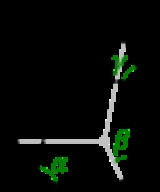Crystal systemEncyclopedia
In crystallography
Crystallography
Crystallography is the experimental science of the arrangement of atoms in solids. The word "crystallography" derives from the Greek words crystallon = cold drop / frozen drop, with its meaning extending to all solids with some degree of transparency, and grapho = write.Before the development of...

, the terms crystal system, crystal family, and lattice system each refer to one of several classes of space group
Space group
In mathematics and geometry, a space group is a symmetry group, usually for three dimensions, that divides space into discrete repeatable domains.In three dimensions, there are 219 unique types, or counted as 230 if chiral copies are considered distinct...

s, lattices, point group
Point group
In geometry, a point group is a group of geometric symmetries that keep at least one point fixed. Point groups can exist in a Euclidean space with any dimension, and every point group in dimension d is a subgroup of the orthogonal group O...

s, or crystal
Crystal
A crystal or crystalline solid is a solid material whose constituent atoms, molecules, or ions are arranged in an orderly repeating pattern extending in all three spatial dimensions. The scientific study of crystals and crystal formation is known as crystallography...

s. Informally, two crystals tend to be in the same crystal system if they have similar symmetries, though there are many exceptions to this.

Crystal systems, crystal families, and lattice systems are similar but slightly different, and there is widespread confusion between them: in particular the trigonal crystal system is often confused with the rhombohedral lattice system, and the term "crystal system" is sometimes used to mean "lattice system" or "crystal family".

Space group
Space group
In mathematics and geometry, a space group is a symmetry group, usually for three dimensions, that divides space into discrete repeatable domains.In three dimensions, there are 219 unique types, or counted as 230 if chiral copies are considered distinct...

s and crystals are divided into 7 crystal systems according to their point group
Point group
In geometry, a point group is a group of geometric symmetries that keep at least one point fixed. Point groups can exist in a Euclidean space with any dimension, and every point group in dimension d is a subgroup of the orthogonal group O...

s, and into 7 lattice systems according to their Bravais lattices. Five of the crystal systems are essentially the same as five of the lattice systems, but the hexagonal and trigonal crystal systems differ from the hexagonal and rhombohedral lattice systems.
The six crystal families are formed by combining the hexagonal and trigonal crystal systems into one hexagonal family, in order to eliminate this confusion.

## Overview

A lattice system is a class of lattices with the same point group. In three dimensions there are seven lattice systems: triclinic, monoclinic, orthorhombic, tetragonal, rhombohedral, hexagonal, and cubic. The lattice system of a crystal or space group is determined by its lattice but not always by its point group.

A crystal system is a class of point groups. Two point groups are placed in the same crystal system if the sets of possible lattice systems of their space groups are the same. For many point groups there is only one possible lattice system,
and in these cases the crystal system corresponds to a lattice system and is given the same name. However, for the five point groups in the trigonal crystal class there are two possible lattice systems for their point groups: rhombohedral or hexagonal. In three dimensions there are seven crystal systems: triclinic, monoclinic, orthorhombic, tetragonal, trigonal, hexagonal, and cubic. The crystal system of a crystal or space group is determined by its point group but not always by its lattice.

A crystal family also consists of point groups and is formed by combining crystal systems whenever two crystal systems have space groups with the same lattice. In three dimensions a crystal family is almost the same as a crystal system (or lattice system), except that the hexagonal and trigonal crystal systems are combined into one hexagonal family. In three dimensions there are six crystal families: triclinic, monoclinic, orthorhombic, tetragonal, hexagonal, and cubic. The crystal family of a crystal or space group is determined by either its point group or its lattice, and crystal families are the smallest collections of point groups with this property.

In dimensions less than three there is no essential difference between crystal systems, crystal families, and lattice systems. There are 1 in dimension 0, 1 in dimension 1, and 4 in dimension 2, called oblique, rectangular, square, and hexagonal.

The relation between three-dimensional crystal families, crystal systems, and lattice systems is shown in the following table:
Crystal family Crystal system Required symmetries of point group point groups
Crystallographic point group
In crystallography, a crystallographic point group is a set of symmetry operations, like rotations or reflections, that leave a central point fixed while moving other directions and faces of the crystal to the positions of features of the same kind...

space group
Space group
In mathematics and geometry, a space group is a symmetry group, usually for three dimensions, that divides space into discrete repeatable domains.In three dimensions, there are 219 unique types, or counted as 230 if chiral copies are considered distinct...

s
bravais lattices Lattice system
Triclinic None 2 2 1 Triclinic
Monoclinic 1 twofold axis of rotation
Rotational symmetry
Generally speaking, an object with rotational symmetry is an object that looks the same after a certain amount of rotation. An object may have more than one rotational symmetry; for instance, if reflections or turning it over are not counted, the triskelion appearing on the Isle of Man's flag has...

or 1 mirror plane
Reflection symmetry
Reflection symmetry, reflectional symmetry, line symmetry, mirror symmetry, mirror-image symmetry, or bilateral symmetry is symmetry with respect to reflection. That is, a figure which does not change upon undergoing a reflection has reflectional symmetry.In 2D there is a line of symmetry, in 3D a...

3 13 2 Monoclinic
Orthorhombic 3 twofold axes of rotation or 1 twofold axis of rotation and two mirror planes. 3 59 4 Orthorhombic
Tetragonal 1 fourfold axis of rotation 7 68 2 Tetragonal
Hexagonal Trigonal 1 threefold axis of rotation 5 7 1 Rhombohedral
18 1 Hexagonal
Hexagonal
Hexagonal crystal system
In crystallography, the hexagonal crystal system is one of the 7 crystal systems, the hexagonal lattice system is one of the 7 lattice systems, and the hexagonal crystal family is one of the 6 crystal families...

1 sixfold axis of rotation 7 27
Cubic
Cubic crystal system
In crystallography, the cubic crystal system is a crystal system where the unit cell is in the shape of a cube. This is one of the most common and simplest shapes found in crystals and minerals....

4 threefold axes of rotation 5 36 3 Cubic
Cubic crystal system
In crystallography, the cubic crystal system is a crystal system where the unit cell is in the shape of a cube. This is one of the most common and simplest shapes found in crystals and minerals....

Total: 6 7 32 230 14 7

## Crystal systems

The distribution of the 32 point groups into the 7 crystal systems is given in the following table.
crystal family crystal system point group
Point group
In geometry, a point group is a group of geometric symmetries that keep at least one point fixed. Point groups can exist in a Euclidean space with any dimension, and every point group in dimension d is a subgroup of the orthogonal group O...

/ crystal class
Schönflies Hermann-Mauguin Orbifold
Orbifold
In the mathematical disciplines of topology, geometry, and geometric group theory, an orbifold is a generalization of a manifold...

Coxeter
Coxeter notation
In geometry, Coxeter notation is a system of classifying symmetry groups, describing the angles between with fundamental reflections of a Coxeter group. It uses a bracketed notation, with modifiers to indicate certain subgroups. The notation is named after H. S. M...

Type order
Symmetry number
The symmetry number or symmetry order of an object is the number of different but indistinguishable arrangements of the object, i.e. the order of its symmetry group...

structure
triclinic
Triclinic crystal system
thumb|180px|Triclinic In crystallography, the triclinic crystal system is one of the 7 crystal systems. A crystal system is described by three basis vectors. In the triclinic system, the crystal is described by vectors of unequal length, as in the orthorhombic system...

triclinic-pedial C1 1 11 [ ]+ enantiomorphic
Chirality (chemistry)
A chiral molecule is a type of molecule that lacks an internal plane of symmetry and thus has a non-superimposable mirror image. The feature that is most often the cause of chirality in molecules is the presence of an asymmetric carbon atom....

polar
Chemical polarity
In chemistry, polarity refers to a separation of electric charge leading to a molecule or its chemical groups having an electric dipole or multipole moment. Polar molecules interact through dipole–dipole intermolecular forces and hydrogen bonds. Molecular polarity is dependent on the difference in...

1 trivial
triclinic-pinacoidal Ci 1x [2,1+] centrosymmetric 2 cyclic
monoclinic
Monoclinic crystal system
In crystallography, the monoclinic crystal system is one of the 7 lattice point groups. A crystal system is described by three vectors. In the monoclinic system, the crystal is described by vectors of unequal length, as in the orthorhombic system. They form a rectangular prism with a...

monoclinic-sphenoidal C2 2 22 [2,2]+ enantiomorphic
Chirality (chemistry)
A chiral molecule is a type of molecule that lacks an internal plane of symmetry and thus has a non-superimposable mirror image. The feature that is most often the cause of chirality in molecules is the presence of an asymmetric carbon atom....

polar
Chemical polarity
In chemistry, polarity refers to a separation of electric charge leading to a molecule or its chemical groups having an electric dipole or multipole moment. Polar molecules interact through dipole–dipole intermolecular forces and hydrogen bonds. Molecular polarity is dependent on the difference in...

2 cyclic
monoclinic-domatic Cs m *11 [ ] polar
Chemical polarity
In chemistry, polarity refers to a separation of electric charge leading to a molecule or its chemical groups having an electric dipole or multipole moment. Polar molecules interact through dipole–dipole intermolecular forces and hydrogen bonds. Molecular polarity is dependent on the difference in...

2 cyclic
monoclinic-prismatic
Prism (geometry)
In geometry, a prism is a polyhedron with an n-sided polygonal base, a translated copy , and n other faces joining corresponding sides of the two bases. All cross-sections parallel to the base faces are the same. Prisms are named for their base, so a prism with a pentagonal base is called a...

C2h 2/m 2* [2,2+] centrosymmetric 4 2×cyclic
orthorhombic
Orthorhombic crystal system
In crystallography, the orthorhombic crystal system is one of the seven lattice point groups. Orthorhombic lattices result from stretching a cubic lattice along two of its orthogonal pairs by two different factors, resulting in a rectangular prism with a rectangular base and height , such that a,...

orthorhombic-sphenoidal D2 222 222 [2,2]+ enantiomorphic
Chirality (chemistry)
A chiral molecule is a type of molecule that lacks an internal plane of symmetry and thus has a non-superimposable mirror image. The feature that is most often the cause of chirality in molecules is the presence of an asymmetric carbon atom....

4 dihedral
orthorhombic-pyramidal
Pyramid (geometry)
In geometry, a pyramid is a polyhedron formed by connecting a polygonal base and a point, called the apex. Each base edge and apex form a triangle. It is a conic solid with polygonal base....

C2v mm2 *22  polar
Chemical polarity
In chemistry, polarity refers to a separation of electric charge leading to a molecule or its chemical groups having an electric dipole or multipole moment. Polar molecules interact through dipole–dipole intermolecular forces and hydrogen bonds. Molecular polarity is dependent on the difference in...

4 dihedral
orthorhombic-bipyramid
Bipyramid
An n-gonal bipyramid or dipyramid is a polyhedron formed by joining an n-gonal pyramid and its mirror image base-to-base.The referenced n-gon in the name of the bipyramids is not an external face but an internal one, existing on the primary symmetry plane which connects the two pyramid halves.The...

al
D2h mmm *222 [2,2] centrosymmetric 8 2×dihedral
tetragonal
Tetragonal crystal system
In crystallography, the tetragonal crystal system is one of the 7 lattice point groups. Tetragonal crystal lattices result from stretching a cubic lattice along one of its lattice vectors, so that the cube becomes a rectangular prism with a square base and height .There are two tetragonal Bravais...

tetragonal-pyramidal C4 4 44 + enantiomorphic
Chirality (chemistry)
A chiral molecule is a type of molecule that lacks an internal plane of symmetry and thus has a non-superimposable mirror image. The feature that is most often the cause of chirality in molecules is the presence of an asymmetric carbon atom....

polar
Chemical polarity
In chemistry, polarity refers to a separation of electric charge leading to a molecule or its chemical groups having an electric dipole or multipole moment. Polar molecules interact through dipole–dipole intermolecular forces and hydrogen bonds. Molecular polarity is dependent on the difference in...

4 Cyclic
tetragonal-disphenoidal S4 2x [2+,2]   4 cyclic
tetragonal-dipyramidal C4h 4/m 4* [2,4+] centrosymmetric 8 2×cyclic
tetragonal-trapezoidal D4 422 422 [2,4]+ enantiomorphic
Chirality (chemistry)
A chiral molecule is a type of molecule that lacks an internal plane of symmetry and thus has a non-superimposable mirror image. The feature that is most often the cause of chirality in molecules is the presence of an asymmetric carbon atom....

8 dihedral
ditetragonal-pyramidal C4v 4mm *44  polar
Chemical polarity
In chemistry, polarity refers to a separation of electric charge leading to a molecule or its chemical groups having an electric dipole or multipole moment. Polar molecules interact through dipole–dipole intermolecular forces and hydrogen bonds. Molecular polarity is dependent on the difference in...

8 dihedral
tetragonal-scalenoidal D2d 2m or m2 2*2 [2+,4]   8 dihedral
ditetragonal-dipyramidal D4h 4/mmm *422 [2,4] centrosymmetric 16 2×dihedral
hexagonal  trigonal trigonal-pyramidal C3 3 33 + enantiomorphic
Chirality (chemistry)
A chiral molecule is a type of molecule that lacks an internal plane of symmetry and thus has a non-superimposable mirror image. The feature that is most often the cause of chirality in molecules is the presence of an asymmetric carbon atom....

polar
Chemical polarity
In chemistry, polarity refers to a separation of electric charge leading to a molecule or its chemical groups having an electric dipole or multipole moment. Polar molecules interact through dipole–dipole intermolecular forces and hydrogen bonds. Molecular polarity is dependent on the difference in...

3 cyclic
rhombohedral S6 (C3i) 3x [2+,3+] centrosymmetric 6 cyclic
trigonal-trapezoidal D3 32 or 321 or 312 322 [3,2]+ enantiomorphic
Chirality (chemistry)
A chiral molecule is a type of molecule that lacks an internal plane of symmetry and thus has a non-superimposable mirror image. The feature that is most often the cause of chirality in molecules is the presence of an asymmetric carbon atom....

6 dihedral
ditrigonal-pyramidal C3v 3m or 3m1 or 31m *33  polar
Chemical polarity
In chemistry, polarity refers to a separation of electric charge leading to a molecule or its chemical groups having an electric dipole or multipole moment. Polar molecules interact through dipole–dipole intermolecular forces and hydrogen bonds. Molecular polarity is dependent on the difference in...

6 dihedral
ditrigonal-scalahedral D3d m or m1 or 1m 2*3 [2+,6] centrosymmetric 12 dihedral
hexagonal
Hexagonal crystal system
In crystallography, the hexagonal crystal system is one of the 7 crystal systems, the hexagonal lattice system is one of the 7 lattice systems, and the hexagonal crystal family is one of the 6 crystal families...

hexagonal-pyramidal C6 6 66 + enantiomorphic
Chirality (chemistry)
A chiral molecule is a type of molecule that lacks an internal plane of symmetry and thus has a non-superimposable mirror image. The feature that is most often the cause of chirality in molecules is the presence of an asymmetric carbon atom....

polar
Chemical polarity
In chemistry, polarity refers to a separation of electric charge leading to a molecule or its chemical groups having an electric dipole or multipole moment. Polar molecules interact through dipole–dipole intermolecular forces and hydrogen bonds. Molecular polarity is dependent on the difference in...

6 cyclic
trigonal-dipyramidal C3h 3* [2,3+]   6 cyclic
hexagonal-dipyramidal C6h 6/m 6* [2,6+] centrosymmetric 12 2×cyclic
hexagonal-trapezoidal D6 622 622 [2,6]+ enantiomorphic
Chirality (chemistry)
A chiral molecule is a type of molecule that lacks an internal plane of symmetry and thus has a non-superimposable mirror image. The feature that is most often the cause of chirality in molecules is the presence of an asymmetric carbon atom....

12 dihedral
dihexagonal-pyramidal C6v 6mm *66  polar
Chemical polarity
In chemistry, polarity refers to a separation of electric charge leading to a molecule or its chemical groups having an electric dipole or multipole moment. Polar molecules interact through dipole–dipole intermolecular forces and hydrogen bonds. Molecular polarity is dependent on the difference in...

12 dihedral
ditrigonal-dipyramidal D3h m2 or 2m *322 [2,3]   12 dihedral
dihexagonal-dipyramidal D6h 6/mmm *622 [2,6] centrosymmetric 24 2×dihedral
cubic
Cubic crystal system
In crystallography, the cubic crystal system is a crystal system where the unit cell is in the shape of a cube. This is one of the most common and simplest shapes found in crystals and minerals....

tetrahedral T 23 332 [3,3]+ enantiomorphic
Chirality (chemistry)
A chiral molecule is a type of molecule that lacks an internal plane of symmetry and thus has a non-superimposable mirror image. The feature that is most often the cause of chirality in molecules is the presence of an asymmetric carbon atom....

12 Alternating
tetrahedral Td 3m *332 [3,3]   24 symmetric
diploidal Th m 3*2 [3+,4] centrosymmetric 24 2×alternating
gyroidal O 432 432 [4,3]+ enantiomorphic
Chirality (chemistry)
A chiral molecule is a type of molecule that lacks an internal plane of symmetry and thus has a non-superimposable mirror image. The feature that is most often the cause of chirality in molecules is the presence of an asymmetric carbon atom....

24 symmetric
hexoctahedral Oh mm *432 [4,3] centrosymmetric 48 2×symmetric

The crystal structure
Crystal structure
In mineralogy and crystallography, crystal structure is a unique arrangement of atoms or molecules in a crystalline liquid or solid. A crystal structure is composed of a pattern, a set of atoms arranged in a particular way, and a lattice exhibiting long-range order and symmetry...

s of biological molecules (such as protein
Protein
Proteins are biochemical compounds consisting of one or more polypeptides typically folded into a globular or fibrous form, facilitating a biological function. A polypeptide is a single linear polymer chain of amino acids bonded together by peptide bonds between the carboxyl and amino groups of...

structures) can only occur in the 11 enantiomorphic
Chirality (chemistry)
A chiral molecule is a type of molecule that lacks an internal plane of symmetry and thus has a non-superimposable mirror image. The feature that is most often the cause of chirality in molecules is the presence of an asymmetric carbon atom....

point groups, as biological molecules are invariably chiral
Chirality (chemistry)
A chiral molecule is a type of molecule that lacks an internal plane of symmetry and thus has a non-superimposable mirror image. The feature that is most often the cause of chirality in molecules is the presence of an asymmetric carbon atom....

. The protein assemblies themselves may have symmetries other than those given above, because they are not intrinsically restricted by the Crystallographic restriction theorem
Crystallographic restriction theorem
The crystallographic restriction theorem in its basic form was based on the observation that the rotational symmetries of a crystal are usually limited to 2-fold, 3-fold, 4-fold, and 6-fold...

RAD52 homolog , also known as RAD52, is a protein which in humans is encoded by the RAD52 gene.- Function :The protein encoded by this gene shares similarity with Saccharomyces cerevisiae Rad52, a protein important for DNA double-strand break repair and homologous recombination...

DNA binding protein has an 11-fold rotational symmetry (in human), however, it must form crystals in one of the 11 enantiomorphic
Chirality (chemistry)
A chiral molecule is a type of molecule that lacks an internal plane of symmetry and thus has a non-superimposable mirror image. The feature that is most often the cause of chirality in molecules is the presence of an asymmetric carbon atom....

point groups given above.

## Lattice systems

The distribution of the 14 Bravais lattice types into 7 lattice systems is given in the following table.
 The 7 lattice systems The 14 Bravais Lattices triclinicTriclinic crystal systemthumb|180px|Triclinic In crystallography, the triclinic crystal system is one of the 7 crystal systems. A crystal system is described by three basis vectors. In the triclinic system, the crystal is described by vectors of unequal length, as in the orthorhombic system... (parallelepipedParallelepipedIn geometry, a parallelepiped is a three-dimensional figure formed by six parallelograms. By analogy, it relates to a parallelogram just as a cube relates to a square. In Euclidean geometry, its definition encompasses all four concepts...)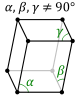monoclinicMonoclinic crystal systemIn crystallography, the monoclinic crystal system is one of the 7 lattice point groups. A crystal system is described by three vectors. In the monoclinic system, the crystal is described by vectors of unequal length, as in the orthorhombic system. They form a rectangular prism with a... (right prismPrism (geometry)In geometry, a prism is a polyhedron with an n-sided polygonal base, a translated copy , and n other faces joining corresponding sides of the two bases. All cross-sections parallel to the base faces are the same. Prisms are named for their base, so a prism with a pentagonal base is called a... with parallelogramParallelogramIn Euclidean geometry, a parallelogram is a convex quadrilateral with two pairs of parallel sides. The opposite or facing sides of a parallelogram are of equal length and the opposite angles of a parallelogram are of equal measure... base; here seen from above) simple base-centered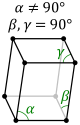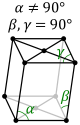orthorhombicOrthorhombic crystal systemIn crystallography, the orthorhombic crystal system is one of the seven lattice point groups. Orthorhombic lattices result from stretching a cubic lattice along two of its orthogonal pairs by two different factors, resulting in a rectangular prism with a rectangular base and height , such that a,... (cuboidCuboidIn geometry, a cuboid is a solid figure bounded by six faces, forming a convex polyhedron. There are two competing definitions of a cuboid in mathematical literature...) simple base-centered body-centered face-centered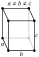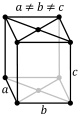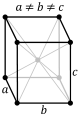tetragonalTetragonal crystal systemIn crystallography, the tetragonal crystal system is one of the 7 lattice point groups. Tetragonal crystal lattices result from stretching a cubic lattice along one of its lattice vectors, so that the cube becomes a rectangular prism with a square base and height .There are two tetragonal Bravais... (square cuboidCuboidIn geometry, a cuboid is a solid figure bounded by six faces, forming a convex polyhedron. There are two competing definitions of a cuboid in mathematical literature...) simple body-centered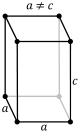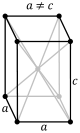rhombohedral (trigonal trapezohedronTrigonal trapezohedronIn geometry, the trigonal trapezohedron or deltohedron is the first in an infinite series of face-uniform polyhedra which are dual to the antiprisms. It has six faces which are congruent rhombi....)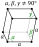hexagonalHexagonal crystal systemIn crystallography, the hexagonal crystal system is one of the 7 crystal systems, the hexagonal lattice system is one of the 7 lattice systems, and the hexagonal crystal family is one of the 6 crystal families... (centered regular hexagon)cubicCubic crystal systemIn crystallography, the cubic crystal system is a crystal system where the unit cell is in the shape of a cube. This is one of the most common and simplest shapes found in crystals and minerals....(isometric; cubeCubeIn geometry, a cube is a three-dimensional solid object bounded by six square faces, facets or sides, with three meeting at each vertex. The cube can also be called a regular hexahedron and is one of the five Platonic solids. It is a special kind of square prism, of rectangular parallelepiped and...) simple body-centered face-centered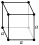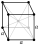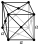In geometry
Geometry
Geometry arose as the field of knowledge dealing with spatial relationships. Geometry was one of the two fields of pre-modern mathematics, the other being the study of numbers ....

and crystallography
Crystallography
Crystallography is the experimental science of the arrangement of atoms in solids. The word "crystallography" derives from the Greek words crystallon = cold drop / frozen drop, with its meaning extending to all solids with some degree of transparency, and grapho = write.Before the development of...

, a Bravais lattice is a category of symmetry group
Symmetry group
The symmetry group of an object is the group of all isometries under which it is invariant with composition as the operation...

s for translational symmetry
Translational symmetry
In geometry, a translation "slides" an object by a a: Ta = p + a.In physics and mathematics, continuous translational symmetry is the invariance of a system of equations under any translation...

in three directions, or correspondingly, a category of translation lattice
Lattice (group)
In mathematics, especially in geometry and group theory, a lattice in Rn is a discrete subgroup of Rn which spans the real vector space Rn. Every lattice in Rn can be generated from a basis for the vector space by forming all linear combinations with integer coefficients...

s.

Such symmetry groups consist of translations by vectors of the form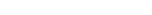where n1, n2, and n3 are integer
Integer
The integers are formed by the natural numbers together with the negatives of the non-zero natural numbers .They are known as Positive and Negative Integers respectively...

s and a1, a2, and a3 are three non-coplanar vectors, called primitive vectors.

These lattices are classified by space group
Space group
In mathematics and geometry, a space group is a symmetry group, usually for three dimensions, that divides space into discrete repeatable domains.In three dimensions, there are 219 unique types, or counted as 230 if chiral copies are considered distinct...

of the translation lattice itself; there are 14 Bravais lattices in three dimensions; each can apply in one lattice system only. They represent the maximum symmetry a structure with the translational symmetry concerned can have.

All crystalline materials must, by definition fit in one of these arrangements (not including quasicrystal
Quasicrystal
A quasiperiodic crystal, or, in short, quasicrystal, is a structure that is ordered but not periodic. A quasicrystalline pattern can continuously fill all available space, but it lacks translational symmetry...

s).

For convenience a Bravais lattice is depicted by a unit cell which is a factor 1, 2, 3 or 4 larger than the primitive cell
Primitive cell
Used predominantly in geometry, solid state physics, and mineralogy, particularly in describing crystal structure, a primitive cell is a minimum cell corresponding to a single lattice point of a structure with translational symmetry in 2 dimensions, 3 dimensions, or other dimensions...

. Depending on the symmetry of a crystal or other pattern, the fundamental domain
Fundamental domain
In geometry, the fundamental domain of a symmetry group of an object is a part or pattern, as small or irredundant as possible, which determines the whole object based on the symmetry. More rigorously, given a topological space and a group acting on it, the images of a single point under the group...

is again smaller, up to a factor 48.

The Bravais lattices were studied by Moritz Ludwig Frankenheim (1801-1869), in 1842, who found that there were 15 Bravais lattices. This was corrected to 14 by A. Bravais
Auguste Bravais
Auguste Bravais was a French physicist, well known for his work in crystallography...

in 1848.

• Crystal cluster
Crystal cluster
A crystal depending upon its chemical composition, bonding power, atmospheric pressure and ambient environment form clusters. A crystal cluster is the development of a crystal system and is also known as a crystal family. Though crystal family as a technical term in crystallography is a matrix of...

• Crystal structure
Crystal structure
In mineralogy and crystallography, crystal structure is a unique arrangement of atoms or molecules in a crystalline liquid or solid. A crystal structure is composed of a pattern, a set of atoms arranged in a particular way, and a lattice exhibiting long-range order and symmetry...

• List of the 230 crystallographic 3D space groups